# Masterminda program for the Texas Instruments TI-83and TI-84 (Plus) graphing calculators

### A Mastermind game based on numerals instead of colors

Play the familiar game Mastermind with numerals instead of colors and have a hunt for a secret number. The random number is generated by your calculator. Available 'colors' are the numerals from 1 to 9.

To find a four digit secret number the first attempt is for instance 1234.
According to the returned response [1 1234 0 2] no numeral is found to be at the right position while two numerals form part of the hidden number at other locations.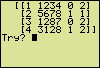The second examination leads to [1 1], meaning one right numeral at the right position and one right numeral at a wrong position. From both responses it follows that the number in demand holds no nines.
After four attempts the possible numbers left are 6318, 2358 and 2368. Getting the desired outcome [4 0] at the next attempt is only a matter of being in luck...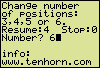This Mastermind game can be played at different levels of difficulty. Just make the program generate a random number with more or less digits. To do so, put in a "0", press Enter more than once to pass through some help screens and select 3, 4, 5 or 6 digits.
The option "Resume" enables you to continue a running game. "Stop" (0) will end the running program (MASTRMND).

In case of a six digit number the game could pass as follows.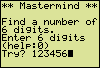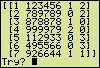The first two outcomes indicate a double or triple presence of a numeral in the unknown number. After seven examinations only four possible numbers remain:
951471 (double 1), 943375 and 963375 (double 3), and 953275 (double 5).
Evaluating 943375 will result in [2 2], [6 0], [5 0] or [4 0], so it's a hit or the final decision is within close reach.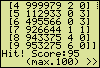Memory overflow
During the course of a game the amount of stored data increases. To prevent a memory overflow error message from spoiling your game, be sure your calculator has sufficient free memory. In most cases 3500 bytes will do (for both program and data). A check of the amount of free memory is only a few keystrokes away:
> 2nd,MEM > Mem Mgmt/Del... > RAM FREE.

Have a nice playing time.

### Program Listing

[ Remarks ]
PROGRAM:MASTRMND
"Mem [A]-[D],IJKZ"
"ver200410c"
[ memories in use, version ]
Normal:Float
[ > MODE > Normal ]
Full
4→K
[ → : STO-key ]

Lbl A0
[ > PRGM > CTL > Lbl ]
iPart(K)→K
[ > MATH > NUM > iPart( ]
min(max(3,K),6)→K
{4,K}→dim([A])
[ > 2nd,MATRIX > MATH > dim( ]
[ > 2nd,MATRIX > NAMES > [A] ]
Fill(1,[A])
[A]+int(.9*abs(randM(4,K)))→[A]
[ > 2nd,MATRIX > MATH > randM( ]
K+2→Z
(ln(396/391)/ln(2))^.5→J
(1–1.5J)/(1–J)*Z→[A](4,1)
(1.5Z–[A](4,1))^2/ln(2)→[A](4,2)
{1,4}→dim([B])
Fill(0,[B])
{7,4}→dim([D])
Lbl A1
ClrHome
[ > PRGM > I/O > ClrHome ]
Disp "** Mastermind **","","Find a number of","  digits."
[ two spaces in front of "digits" ]
Disp "Enter   digits","(help:0)"
[ 3 spaces between "Enter" and "digits" ]
Output(4,1,K)
Output(5,7,K)
Lbl A2
0→Z
Input "Try? ",Z
abs(Z)→Z
If Z<.1*10^K
[ > 2nd,TEST > TEST > "<" ]
[ mark the decimal point ]
Goto C0
(Z–fPart(Z))/10^K→Z
Z–int(Z)→Z
Z*10^K→[B](1,2)
0→[B](1,3)
0→[B](1,4)
Z→[A](4,3)
*row(0,[A],1)→[A]
[ > 2nd,MATRIX > MATH > *row( ]
row+([A],3,1)→[A]
0→J
Lbl A3
J+1→J
10[A](4,3)→[A](4,3)
int([A](4,3))→[A](2,J)
If [A](2,J)=0
Goto C0
[A](4,3)–[A](2,J)→[A](4,3)
If J<K
Goto A3
0→J

Lbl B0
J+1→J
J→I
If [A](1,J)≠[A](2,I)
Goto B1
[B](1,3)+1→[B](1,3)
-1→[A](1,J)
[ negation symbol (-),
[ not subtraction symbol ]
-2→[A](2,I)
Lbl B1
If J<K
Goto B0
0→J

Lbl B2
J+1→J
0→I
If [A](1,J)=-1
Goto B5
Lbl B3
I+1→I
If [A](1,J)≠[A](2,I)
Goto B4
[B](1,4)+1→[B](1,4)
-1→[A](1,J)
-2→[A](2,I)
K→I
Lbl B4
If I<K
Goto B3
Lbl B5
If J<K
Goto B2

[B](1,1)+1→[B](1,1)
If [B](1,1)=1
Then
[B]→[C]
Else
(augment([C]T,[B]T))T→[C]
[ > 2nd,MATRIX > MATH > T ]
End
ClrHome
If [B](1,1)<8
Then
Disp [C]
Else
[B](1,1)–7→Z
For(I,1,7)
For(J,1,4)
[C](Z+I,J)→[D](I,J)
End
End
Disp [D]
End
If [B](1,3)<K
Goto A2
Disp ""
Output(7,1,"Hit! Score:")
[A](4,1)–[B](1,1)→Z
e^(Z*abs(Z)/[A](4,2))→Z
[ e^( : 2nd,ex ]
min(Z,1)→Z
([B](1,3)+.7[B](1,4))/K→J
10+90*(.56J+.44Z)*J→Z
int((Z+.5)/5)*5→Z
Output(7,12,Z)
Output(8,5,"(max.100) >>")
Pause
Goto A0

Lbl C0
ClrHome
Disp "Valid","digits: 1-9",""
Disp "Invalid attempts","(examples)","3303, 0003, 3"
Output(8,1,">>")
Pause
ClrHome
Disp "Response:"
Disp "1 Attempt number"
Disp "  Numbr of right","  digits at"
[ 2 spaces in front of "Numbr" and "digits" ]
Disp "3 right position"
Disp "4 wrong position"
Output(8,1,">>")
Pause
ClrHome
Disp "Change number","of positions:","3,4,5 or 6"
Disp "Resume:   Stop:0"
[ 3 spaces between "Resume:" and "Stop" ]
Output(4,8,K)
Output(7,1,"info:")
Output(8,1,"www.tenhorn.com")
Input "Number? ",Z
ClrHome
If Z=0
Then
DelVar [A]
DelVar [B]
DelVar [C]
DelVar [D]
Stop
End
If (Z=K) and ([B](1,1)>0)
[ > 2nd,TEST > LOGIC > and ]
Then
If [B](1,1)<8
Then
Disp [C]
Else
Disp [D]
End
Goto A2
End
Z→K
Goto A0

If you need help with programming your calculator, this exercise will put you on the way: Creating a Program for the TI-83 calculator

Hein ten Horn
Top | Programs Texas Instruments | Home